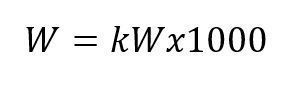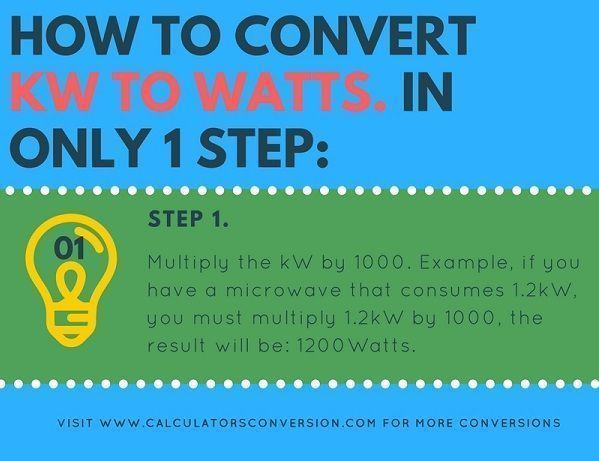# kW to Watts – Convert automatically, formula, calculator, chart (table)

With this tool you can convert online kW to Watts automatically, easy, fast and free.

For greater ease we explain that formula is used for the calculation and a table with the main conversions of kW to Watts. Also show how to convert kW to Watts in one step

## kW to Watts calculation formula 3phase, 2 phase, 1 phase:• Watts=Watt is the unit of power (symbol: W).
• kW=One kilowatt is equal to 1000 watts.

## How to convert kW to Watts in only 1 step:## Step 1:

Multiply the kW by 1000. Example, if you have a microwave that consumes 1.2kW, you must multiply 1.2kW by 1000, the result will be: 1200Watts.

## Definition kW and Watts:

Watts: is Working Power (also called Actual Power or Active Power or Real Power). It is the power that actually powers the equipment and performs useful work.

The real power in watts is the power that performs work or generates heat. Power in watts is the rate at which energy is consumed (or generated). One watt is one joule (energy) per second (1 W = 1 J/s).

Resistive devices or loads such as heaters, incandescent lamps are rated in Watts.

kW: One kilowatt is equal to 1000 watts.

## kW to Watts table for conversion, equivalence, transformation:

 kW Watts 1 kW 1000 Watts 2 kW 2000 Watts 3 kW 3000 Watts 4 kW 4000 Watts 5 kW 5000 Watts 6 kW 6000 Watts 7 kW 7000 Watts 8 kW 8000 Watts 9 kW 9000 Watts 10 kW 10000 Watts 20 kW 20000 Watts 30 kW 30000 Watts 40 kW 40000 Watts 50 kW 50000 Watts 60 kW 60000 Watts 70 kW 70000 Watts 80 kW 80000 Watts 90 kW 90000 Watts 100 kW 100000 Watts 200 kW 200000 Watts 300 kW 300000 Watts 400 kW 400000 Watts 500 kW 500000 Watts 600 kW 600000 Watts 700 kW 700000 Watts 800 kW 800000 Watts 900 kW 900000 Watts 1000 kW 1000000 Watts 2000 kW 2000000 Watts 3000 kW 3000000 Watts 4000 kW 4000000 Watts 5000 kW 5000000 Watts 6000 kW 6000000 Watts 7000 kW 7000000 Watts 8000 kW 8000000 Watts 9000 kW 9000000 Watts 10000 kW 10000000 Watts

Rate this conversion kW to Watts:  [kkstarratings]